Question

2. 3: Risk and Rates of Return: Risk in Portfolio Context Risk and Rates of Return:...

2. 3: Risk and Rates of Return: Risk in Portfolio Context Risk and Rates of Return: Risk in Portfolio Context The capital asset pricing model (CAPM) explains how risk should be considered when stocks and other assets are held . The CAPM states that any stock's required rate of return is the risk-free rate of return plus a risk premium that reflects only the risk remaining diversification. Most individuals hold stocks in portfolios. The risk of a stock held in a portfolio is typically the stock's risk when it is held alone. Therefore, the risk and return of an individual stock should be analyzed in terms of how the security affects the risk and return of the portfolio in which it is held. The expected rate of return on a portfolio equals the weighted average of the expected returns on the assets held in the portfolio. A portfolio's risk calculated as the weighted average of the individual stock's standard deviations; the portfolio's risk is generally because diversification the portfolio's risk. Two important terms when discussing are correlation and correlation coefficient. Correlation is the tendency of two variables to move together, while correlation coefficient is a measure of the degree of relationship between two variables. If a portfolio consists of two stocks that are perfectly correlated then the portfolio is riskless because the stocks' returns move countercyclically to each other. If the returns of the stocks are perfectly correlated then the stocks' returns would move up and down together and the portfolio would be exactly as risky as the individual stocks. In this situation, diversification would be completely for reducing risk. In reality, most stocks are correlated but not perfectly. So, combining stocks into portfolios reduces risk but does not completely eliminate it. This illustrates that can reduce risk, but not completely eliminate risk. Portfolios risk can be broken down into two types. risk is that part of a security's risk associated with random events. It can be eliminated by proper diversification and is also known as company-specific risk. On the other hand, risk is the risk that remains in a portfolio after diversification has eliminated all company-specific risk. Standard deviation is not a good measure of risk when a stock is held in a portfolio. A stock's relevant risk is the risk that remains once a stock is in a diversified portfolio. Its contribution to the portfolio's market risk is measured by a stock's , which shows the extent to which a given stock's returns move up and down with the stock market. An average stock's beta is 1 because an average-risk stock is one that tends to move up and down in step with the general market. A stock with a beta 1 is considered to have high risk, while a stock with beta 1 is considered to have low risk. Quantitative Problem: You are holding a portfolio with the following investments and betas: Stock Dollar investment Beta A \$300,000 1.35 B 100,000 1.5 C 300,000 0.65 D 300,000 -0.3 Total investment \$1,000,000 The market's required return is 10% and the risk-free rate is 5%. What is the portfolio's required return? Round your answer to 3 decimal places. Do not round intermediate calculations. %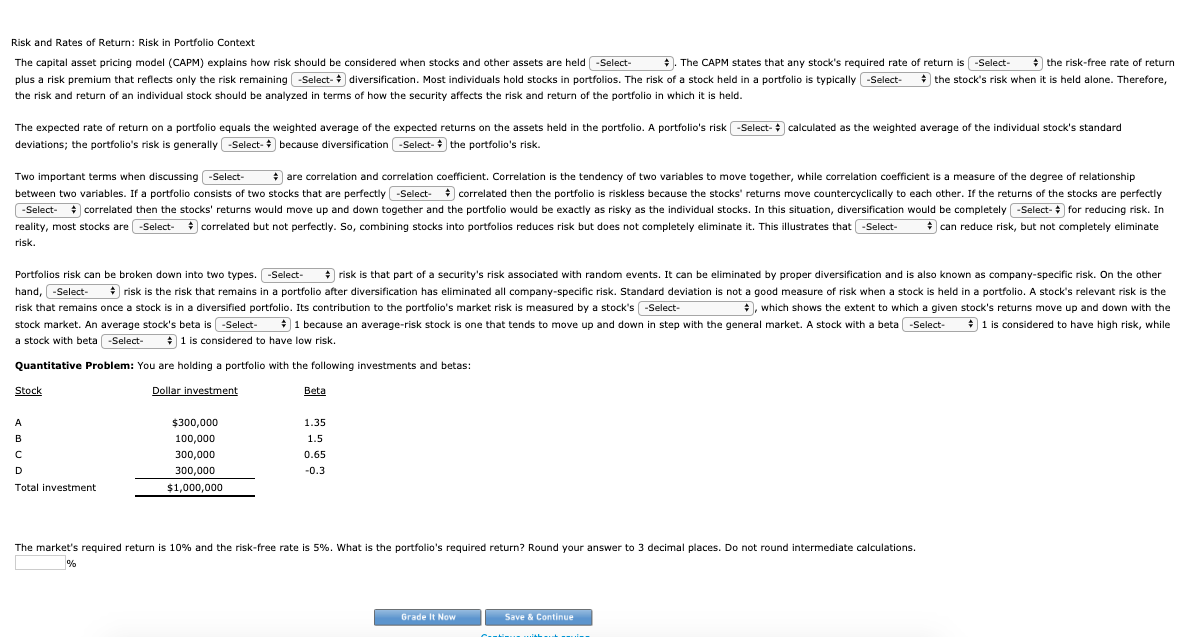Risk and Rates of Return: Risk in Portfolio Context The capital asset pricing model (CAPM) explains how risk should be considered when stocks and other assets are held -Select- The CAPM states that any stock's required rate of return is -Select the risk-free rate of return plus a risk premium that reflects only the risk remaining -Select- diversification. Most individuals hold stocks in portfolios. The risk of a stock held in a portfolio is typically -Select the stock's risk when it is held alone. Therefore, the risk and return of an individual stock should be analyzed in terms of how the security affects the risk and return of the portfolio in which it is held. -Select- calculated as the weighted average of the individual stock's standard The expected rate of return on a portfolio equals the weighted average of the expected returns on the assets held in the portfolio. A portfolio's risk deviations; the portfolio's risk is generally -Select- because diversification - Select the portfolio's risk. Two important terms when discussing -Select- are correlation and correlation coefficient. Correlation is the tendency of two variables to move together, while correlation coefficient is a measure of the degree of relationship between two variables. If a portfolio consists of two stocks that are perfectly -Select correlated then the portfolio is riskless because the stocks' returns move countercyclically to each other. If the returns of the stocks are perfectly -Select- correlated then the stocks' returns would move up and down together and the portfolio would be exactly as risky as the individual stocks. In this situation, diversification would be completely -Select- for reducing risk. In reality, most stocks are -Select- correlated but not perfectly. So, combining stocks into portfolios reduces risk but does not completely eliminate it. This illustrates that -Select- can reduce risk, but not completely eliminate risk. Portfolios risk can be broken down into two types. -Select- risk is that part of a security's risk associated with random events. It can be eliminated by proper diversification and is also known as company-specific risk. On the other hand, -Select- risk is the risk that remains in a portfolio after diversification has eliminated all company-specific risk. Standard deviation is not a good measure of risk when a stock is held in a portfolio. A stock's relevant risk is the risk that remains once a stock is in a diversified portfolio. Its contribution to the portfolio's market risk is measured by a stock's -Select- , which shows the extent to which a given stock's returns move up and down with the stock market. An average stock's beta is -Select- 1 because an average-risk stock is one that tends to move up and down in step with the general market. A stock with a beta -Select- 1 is considered to have high risk, while a stock with beta -Select- 1 is considered to have low risk. Quantitative Problem: You are holding a portfolio with the following investments and betas: Stock Dollar investment Beta \$300,000 100,000 300,000 300,000 \$1,000,000 1.35 1.5 0.65 -0.3 Total investment The market's required return is 10% and the risk-free rate is 5%. What is the portfolio's required return? Round your answer to 3 decimal places. Do not round intermediate calculations. % Grade It Now Save & Continue

Step-1, The Beta of the portfolio

 Stocks Amount invested (\$) Weight to total value [Amount invested / Total value] Beta of the stock Overall Beta [Beta of the stock x Weight to total value] A 3,00,000 0.30 1.35 0.4050 B 1,00,000 0.10 1.50 0.1500 C 3,00,000 0.30 0.65 0.1950 D 3,00,000 0.30 -0.30 -0.0900 TOTAL 10,000,000 1.00 0.6600

Step-2, Portfolio’s required return

As per Capital Asset Pricing Model [CAPM], the required return is calculated by using the following equation

Required rate of return = Risk-free Rate + Beta(Market Rate of Return – Risk-free Rate)

= Rf + B[Rm – Rf]

= 5.000% + 0.66[10.000% - 5.000%]

= 5.000% + [0.66 x 5.000%]

= 5.000% + 3.000%

= 8.300%

“Hence, the Portfolio’s required return will be 8.300%”

Earn Coins

Coins can be redeemed for fabulous gifts.

Similar Homework Help Questions
• od The capital asset pricing model (CAPM) explains how risk should be considered when stocks and...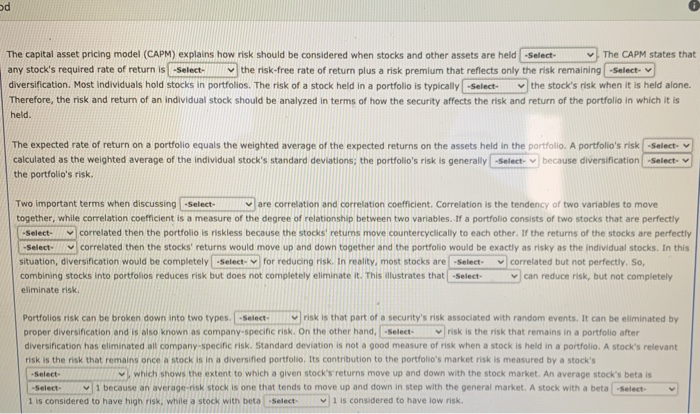od The capital asset pricing model (CAPM) explains how risk should be considered when stocks and other assets are held -Select- The CAPM states that any stock's required rate of return is -Select the risk-free rate of return plus a risk premium that reflects only the risk remaining -Select- diversification. Most individuals hold stocks in portfolios. The risk of a stock held in a portfolio is typically -Select the stock's risk when it is held alone. Therefore, the risk and...

• 2. Portfolio risk and diversification A financial planner is examining the portfolios held by several of...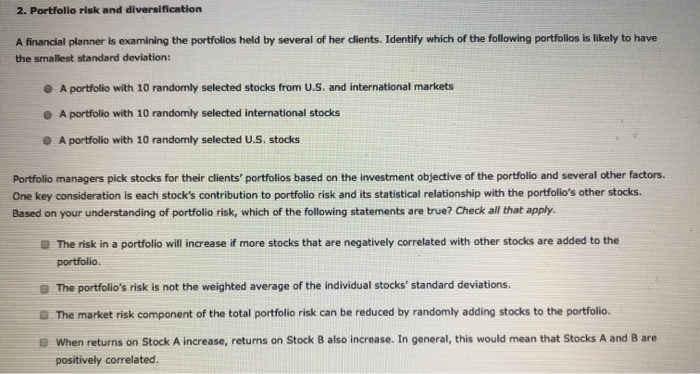2. Portfolio risk and diversification A financial planner is examining the portfolios held by several of her clients. Identify which of the following portfolios is likely to have the smallest standard deviation: A portfolio with 10 randomly selected stocks from U.S. and international markets A portfolio with 10 randomly selected international stocks A portfolio with 10 randomly selected U.S. stocks Portfolio managers pick stocks for their clients' portfolios based on the investment objective of the portfolio and several other factors....

• 3. Portfolio risk and diversification Aa Aa A financial planner is examining the portfolios held by...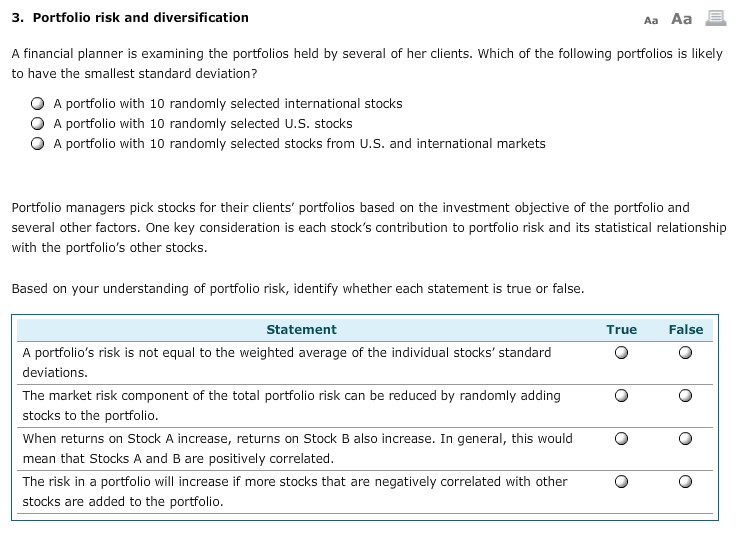3. Portfolio risk and diversification Aa Aa A financial planner is examining the portfolios held by several of her clients. Which of the following portfolios is likely to have the smallest standard deviation ? A portfolio with 10 randomly selected international stocks A portfolio with 10 randomly selected U.S. stocks A portfolio with 10 randomly selected stocks from U.S. and international markets Portfolio managers pick stocks for their clients' portfolios based on the investment objective of the portfolio and several...

• 3. Portfolio risk and diversification Aa Aa E A financial planner is examining the portfolios held...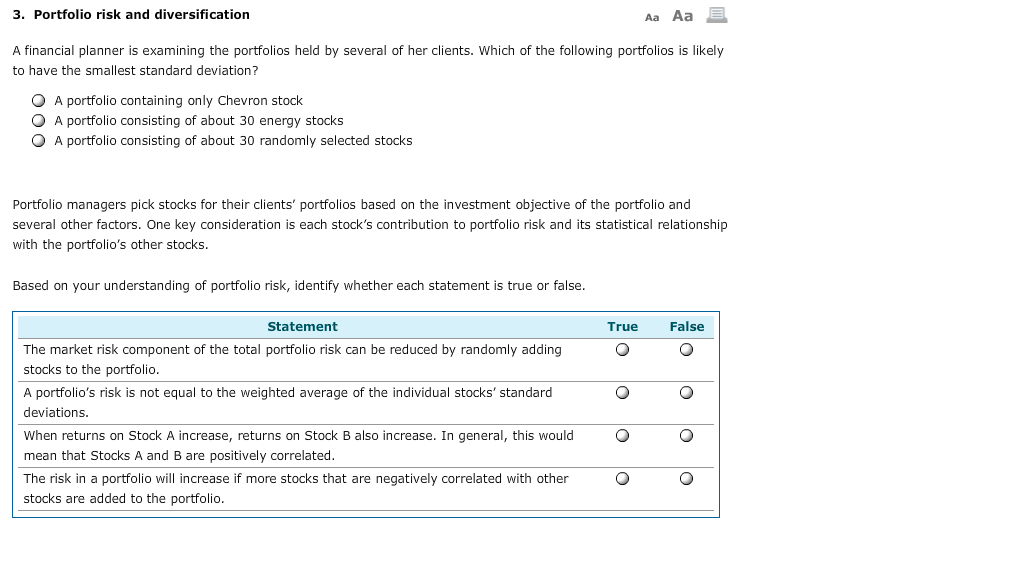3. Portfolio risk and diversification Aa Aa E A financial planner is examining the portfolios held by several of her clients. Which of the following portfolios is likely to have the smallest standard deviation? O O O A portfolio containing only Chevron stock A portfolio consisting of about 30 energy stocks A portfolio consisting of about 30 randomly selected stocks Portfolio managers pick stocks for their clients' portfolios based on the investment objective of the portfolio and several other factors....

• 3. Portfolio risk and diversification A Aa A financial planner is examining the portfolios held by several of her c...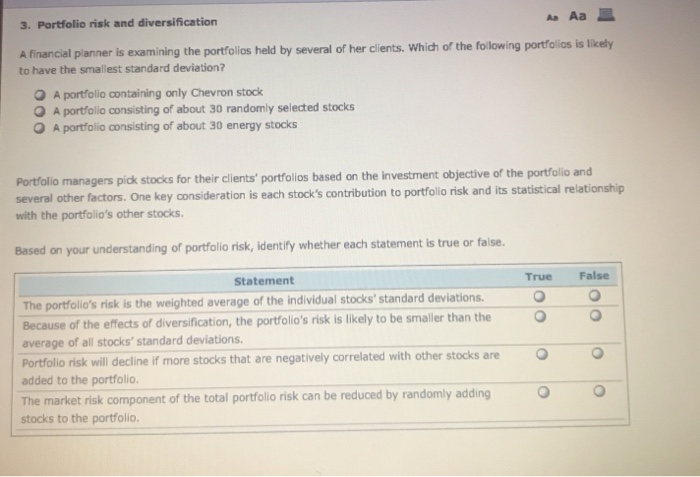3. Portfolio risk and diversification A Aa A financial planner is examining the portfolios held by several of her clients. Which of the following portfolios is likely to have the smallest standard deviation? A portfolio containing only Chevron stock A portfolio consisting of about 30 randomly selected stocks A portfolio consisting of about 30 energy stocks Portfolio managers pick stocks for their clients' portfolios based on the investment objective of the portfolio and several other factors. One key consideration is...

• 6. Portfolio risk and diversification A financial planner is examining the portfolios held by several of...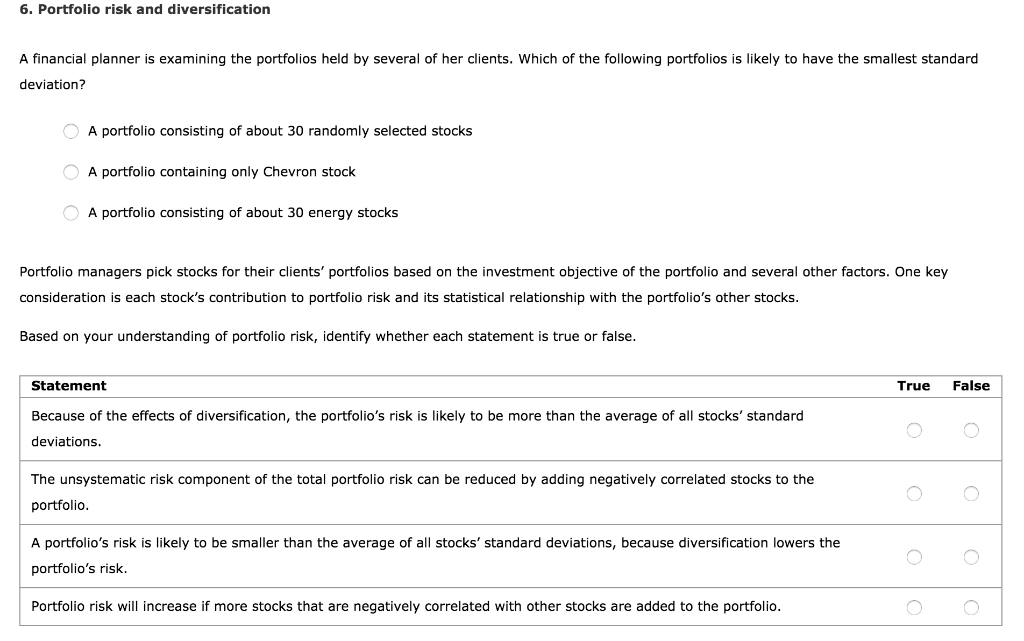6. Portfolio risk and diversification A financial planner is examining the portfolios held by several of her clients. Which of the following portfolios is likely to have the smallest standard deviation? A portfolio consisting of about 30 randomly selected stocks A portfolio containing only Chevron stock A portfolio consisting of about 30 energy stocks Portfolio managers pick stocks for their clients' portfolios based on the investment objective of the portfolio and several other factors. One key consideration is each stock's...

• Assume you wish to evaluate the risk and return behaviors associated with various combinations of two​ stocks, Alpha Sof...

Assume you wish to evaluate the risk and return behaviors associated with various combinations of two​ stocks, Alpha Software and Beta​ Electronics, under three possible degrees of​ correlation: perfect​ positive, uncorrelated, and perfect negative. The average return and standard deviation for each stock appears​ here: Asset   Average Return,overbar r                   Risk (Standard Deviation), s Alpha   5.1%   30.3% Beta                    11.2%      50.5% a. If the returns of assets Alpha and Beta are perfectly positively correlated​ (correlation coefficient equals plus 1​),...

• A financial planner is examining the portfolios held by several of her clients. Which of the...

A financial planner is examining the portfolios held by several of her clients. Which of the following portfolios is likely to have the smallest standard deviation? a.) A portfolio's containing Microsoft, Apple, and Google stock b.) A portfolio consisting of about three randomly selected stocks from different sectors. c.) A portfolio containing only Microsoft stock. Portfolio managers pick stocks for their clients' portfolios based on the investment objective of the portfolio and several other factors. One key consideration is each...

• Please answer Which of the following statements about portfolio diversifications are correct? Check all that apply....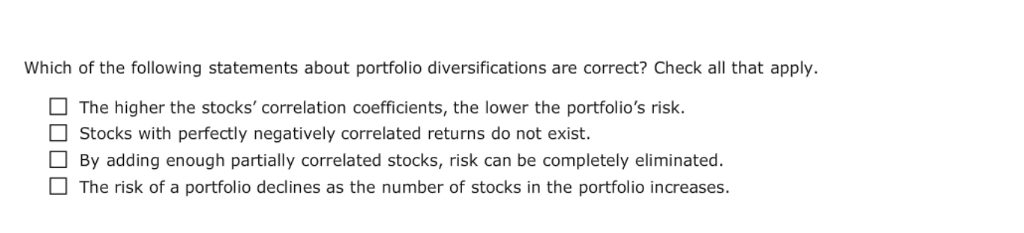Please answer Which of the following statements about portfolio diversifications are correct? Check all that apply. The higher the stocks' correlation coefficients,the lower the portfolio's risk. Stocks with perfectly negatively correlated returns do not exist. By adding enough partially correlated stocks, risk can be completely eliminated The risk of a portfolio declines as the number of stocks in the portfolio increases.

• Using historical data to measure portfolio risk and correlation coefficient Carlos is an investor who believes...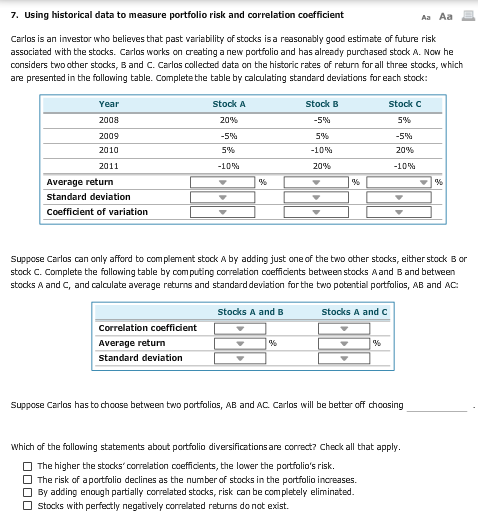Using historical data to measure portfolio risk and correlation coefficient Carlos is an investor who believes that past variability of stocks isa reasonably good estimate of future risk associated with the stocks. Carlos works on creating a new portfolio and has already purchased stock A. Now he considers tv.'o ether stocks, B and C. Carlos collected data on the historic rates of return for all three stocks, which are presented in the following table. Complete the table by calculating standard...